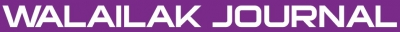Fractional Homotopy Analysis Transforms Method for Solving a Fractional Heat-Like Physical Model

Mohamed KHADER, Sunil KUMAR, Saeid ABBASBANDY

Abstract

The main aim of the present work is to present a new and simple algorithm for time fractional heat like physical models by using the new fractional homotopy analysis transform method (FHATM). The proposed method is an innovative adjustment in the Laplace transform algorithm (LTA) for fractional partial differential equations and makes the calculation much simpler. The numerical solutions obtained by the proposed method indicate that the approach is easy to implement and computationally very attractive. A good agreement between the obtained solution and some well-known results has been obtained.

Keywords

Heat equation, Fractional derivatives, Analytical solution, Mittag-Leffler function, Laplace transform method, fractional homotopy analysis transform method

PDF

References

KB Oldham and J Spanier. The Fractional Calculus: Integrations and Differentiations of Arbitrary Order. Academic Press, New York, 1974.

KS Miller B Ross. An Introduction to the Fractional Calculus and Fractional Differential Equations. Wiley, New York, 1993.

I Podlubny. Fractional Differential Equations. Academic Press, New York, 1999.

K Diethelm. An algorithm for the numerical solution of differential equations of fractional order. Electron Trans. Numer. Anal. 1997; 5, 1-6.

AM Wazwaz and A Gorguis. Exact solutions for heat-like and wave-like equations with variable coefficients. Appl. Math. Comput. 2004; 149, 15-29.

DH Shou and JH He. Beyond Adomian method: The variational iteration method for solving heat-like and wave-like equations with variable coefficients. Phys. Lett. A 2008; 372, 233-7.

T Özis and D Agırseven. He’s homotopy perturbation method for solving heat-like and wave-like equations, with variable coefficients. Phys. Lett. A 2008; 372, 5944-50.

A Sadighi, DD Ganji, M Gorji and N Tolou. Numerical simulation of heat-like models with variable coefficients by the variational iteration method. J. Phys. Conf. Series 2008; 96, 012083.

SJ Liao. 1992. The Proposed Homotopy Analysis Technique for the Solution of Nonlinear Problems. Ph.D. Thesis, Shanghai Jiao Tong University, China.

SJ Liao. Beyond Perturbation: Introduction to the Homotopy Analysis Method. CRC Press, Chapman and Hall, Boca Raton, 1992.

SJ Liao. On the homotopy analysis method for nonlinear problems. Appl. Math. Comput. 2004; 147, 499-513.

SJ Liao. Homotopy analysis method: A new analytical technique for nonlinear problems. Commun. Nonlinear Sci. Numer. Simulat. 1997; 2, 95-100.

SJ Liao and Y Tan. A general approach to obtain series solutions of nonlinear differential equations. Stud. Appl. Math. 2007; 119, 297-355.

K Vishal, S Kumar and S Das. Application of homotopy analysis method for fractional swift Hohenberg equation-Revisited. Appl. Math. Modell. 2012; 36, 3630-7.

H Jafari, A Golbabai, S Seifi and K Sayevand. Homotopy analysis method for solving multi-term linear and nonlinear diffusion wave equations of fractional order. Comput. Math. Appl. 2010; 59, 1337-44.

X Zhang, B Tang and Y He. Homotopy analysis method for higher-order fractional integro-differential equations. Comput. Math. Appl. 2011; 62 3194-203.

RK Pandey, OP Singh and VK Baranwal. An analytic algorithm for the space-time fractional advection-dispersion equation. Comput. Phys. Commun. 2011; 182, 1134-44.

NA Khan, M Jamil and A Ara. Approximate solution of time fractional Schrodinger equation via homotopy analysis method. ISRN Math. Phys. 2012; 2012, 197068.

AR Ghotbi H Bararnia, G Domairry and A Barari. Investigation of powerful analytical method in to natural convection boundary layer flow. Commun. Nonlinear Sci. Numer. Simulat. 2009; 15, 2222-8.

S Abbasbandy, E Shivanian and K Vajravelu. Mathematical properties of h-curve in the frame work of the homotopy analysis method. Commun. Nonlinear Sci. Numer. Simulat. 2011; 16, 4268-75.

S Abbasbandy. Homotopy analysis method for the Kawahara equation. Nonlin. Anal. Real World Appl. 2010; 11, 307-12.

S Abbasbandy. Approximate solution for the nonlinear model of diffusion and reaction in Porous catalysts by means of the homotopy analysis method. Chem. Eng. J. 2008; 136, 144-50.

NH Sweilam and MM Khader. Semi exact solutions for the bi-harmonic equation using homotopy analysis method. World Appl. Sci. J. 2011; 13, 1-7.

AM Wazwaz. The combined Laplace transform-Adomian decomposition method for handling nonlinear Volterra integro-differential equations. Appl. Math. Comp. 2010; 216, 1304-9.

M Khan, MA Gondal and S Kumar. A new analytical procedure for nonlinear integral equation. Math. Comput. Modell. 2012; 55, 1892-7.

Y Khan, N Faraz, S Kumar and A Yildirim. A coupling method of homotopy perturbation and Laplace transform for fractional models. U.P.B. Sci. Bull. Series A 2012; 74, 57-68.

S Kumar, H Kocak and A Yildirim. A fractional model of gas dynamics equation and its approximate solution by using Laplace transform. Z. Naturforsch 2012; 67, 389-96.

M Khan, MA Gondal, I Hussain and SK Vanani. A new comparative study between homotopy analysis transform method and homotopy perturbation transform method on a semi-infinite domain. Math. Comput. Modell. 2012; 55, 1143-50.

VG Gupta and S Gupta. Applications of homotopy analysis transform method for solving various nonlinear equations. World Appl. Sci. J. 2012; 18, 1839-46.

MA Gonda, AS Arife, M Khan and I Hussain. An efficient numerical method for solving linear and nonlinear partial differential equations by combining homotopy analysis and transform method. World Appl. Sci. J. 2012; 14, 1786-91.

MS Mohamed, F Al-Malki and M Al-humyani. Homotopy analysis transform method for time-space fractional gas dynamics equation. Gen. Math. Notes 2014; 24, 1-6.

Y Luchko and R Gorenflo. An operational method for solving fractional differential equations with the Caputo derivatives. Acta Math. Vietnamica 1999; 24, 207-33.

OL Moustafa. On the Cauchy problem for some fractional order partial differential equations. Chaos Soliton. Fract. 2003; 18, 135-40.

G Samko, AA Kilbas and OI Marichev. Fractional Integrals and Derivatives: Theory and Applications. Gordon and Breach. Yverdon, 1993.

F Mainardi. On the Initial Value Problem for the Fractional Diffusion-Wave Equation. In: S Rionero and T Ruggeeri (eds.). Waves and Stability in Continuous Media, World Scientific, Singapore, 1994, p. 246-56.

MM Khader, S Kumar and S Abbasbandy. New homotopy analysis transform method for solving the discontinued problems arising in nanotechnology. Chin. Phys. B 2013; 22, 110201.

Refbacks

• There are currently no refbacks.Online ISSN: 2228-835X

http://wjst.wu.ac.th

Last updated: 17 May 2019